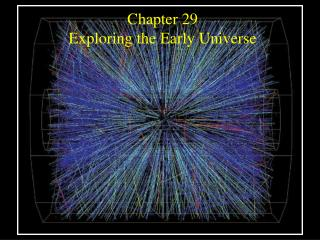DownloadDownload PresentationChapter 29 Exploring the Early Universe

# Chapter 29 Exploring the Early Universe

Télécharger la présentation## Chapter 29 Exploring the Early Universe

- - - - - - - - - - - - - - - - - - - - - - - - - - - E N D - - - - - - - - - - - - - - - - - - - - - - - - - - -
##### Presentation Transcript

1. Chapter 29 Exploring the Early Universe

2. Guiding Questions • Has the universe always expanded as it does today? • What is antimatter? How can it be created, and how is it destroyed? • Why is antimatter so rare today? • What materials in today’s universe are remnants of nuclear reactions in the hot early universe? • How did the first galaxies form? • How were the fundamental forces of nature different during the first second after the Big Bang? • Are scientists close to developing an all-encompassing “theory of everything”? • How many dimensions do unified models predict?

3. The Isotropy or Horizon problem: why should the universe look the same in all directions? The Flatness problem: why should the density of the universe be just barely enough to prevent recollapse?

4. Before the first second, the universe may have expanded 1050 times in 10-24 s.

5. The newborn universe may have undergone a brief period of vigorous expansion. • PROBLEM: If the universe is at least 26 billion light years across (13 billion in each direction), how could both sides have exactly the same temperature if they couldn’t “communicate?” • SOLUTION: During the first second, the universe underwent a rapid but even expansion, called the inflationary epoch, in which it became many times larger than its original size. Inflation also explains the flatness problem.

6. During inflation, all the mass and energy in the universe burst forth from the vacuum of space. • Quantum mechanics explains the behavior of nature on the atomic scale and smaller. • QM tell us how to calculate the structure of atoms and interactions between atomic nuclei. • Elementary particle physics is the branch of QM that explains how subatomic particles interact. • Heisenberg’s uncertainty principle: one cannot know the exact position and momentum (mass and velocity) of a particle simultaneously. • In cosmology, we cannot simultaneously know the energy precisely for every moment in time.

7. During inflation, all the mass and energy in the universe burst forth from the vacuum of space. • Over brief intervals of time, it is impossible to know exactly how much energy or matter exists in a particular space. • Virtual pairs of particles and antiparticles can virtually exist momentarily

8. As the primordial fireball cooled off, most of the matter and antimatter in the early universe annihilated each other. These pairs of particles are spontaneously created, and then usually annihilate each other.

9. Why didn’t all matter annihilate with antimatter? 1 proton survived for every billion protons annihilated with antiprotons: an example of “symmetry breaking”

10. Nucleosynthesis during the first few minutes of the Big Bang created most of the Helium atoms in the universe.

11. Galaxies formed from density fluctuations in the early universe. Clumps bigger than the Jeans length can grow. LJ = [pkT/mGrm]½ k = 1.38x10-23 J/K T = gas temperature m = mass of one particle of gas G = universal gravitation constant rm = average density of matter in the gas

12. In grand unified theories, all physical forces had the same strength immediately after the Big Bang. • Gravitational force - attraction between any two objects with mass. • Electromagnetic force - attraction/repulsion between charges, and forces from currents and magnetic fields • Strong nuclear force - holds protons in the nucleus of an atom together. • Weak nuclear force - governs the radioactive decay of neutrons into protons. A theory that correctly combines all forces in the early universe is known as a supergrand unification theory or theory of everything (TOE).

13. GUT unifies 3 out of 4 forces. Standard model explains electroweak unification (2/4).

14. Relative strengths of four fundamental forces in the present time: Gravity is the weakest.

15. Theories attemting to unify the physical forces predict that the universe may have 11 dimensions. • We experience 3 spatial dimensions (up/down, right/left, forward/back). • Plus one time dimension: 3x + 1t = 4 D • Kaluza-Klein theory uses multiple dimensions to describe electricity and magnetism. • String theorists we use 11 D to describe the universe 10x + 1 t = 11 D

16. Guiding Questions • Has the universe always expanded as it does today? • What is antimatter? How can it be created, and how is it destroyed? • Why is antimatter so rare today? • What materials in today’s universe are remnants of nuclear reactions in the hot early universe? • How did the first galaxies form? • How were the fundamental forces of nature different during the first second after the Big Bang? • Are scientists close to developing an all-encompassing “theory of everything”? • How many dimensions do unified models predict?